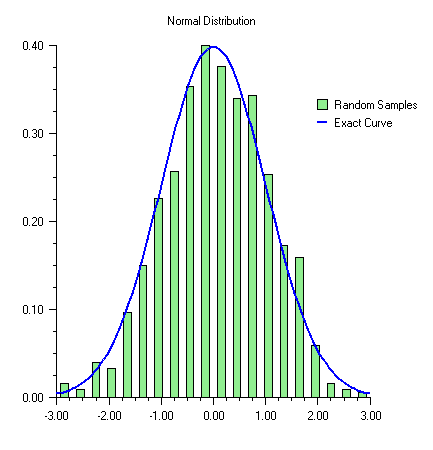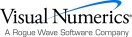## Histogram

A histogram is a bar chart in which the height of the bars is proportional to the frequencies. A histogram generally uses the same axis style as a scatter plot (i.e. the bars are numbered not labeled.)

In IMSL C# Numerical Library, histograms are drawn using the Bar class, but its SetLabels method is not used.

### Example

In this example normally distributed random numbers are generated and placed into 20 uniformly sized bins in the interval [-3,3]. Points outside of this interval are ignored. The bin counts are scaled by the number of samples and the bin width. The scaled bin counts are charted using Bar chart. The exact normal distribution is implemented as a ChartFunction and plotted.

The Legend is displayed by setting the legend node’s IsVisible property to true and defining the bar chart’s Title attribute. The Legend is positioned on the chart by setting its Viewport attribute.using Imsl.Chart2D;

using System;

using System.Drawing;

using Imsl.Stat;

public class SampleHistogram : FrameChart {

public SampleHistogram() {

int nSamples = 1000;

int nBins = 20;

// Setup the bins

double[] bins = new double[nBins];

double dx = 6.0/nBins;

double[] x = new double[nBins];

for (int k = 0; k < x.Length; k++) {

x[k] = -3.0 + (k+0.5)*dx;

}

Imsl.Stat.Random r = new Imsl.Stat.Random(123457);

for (int k = 0; k < nSamples; k++) {

double t = r.NextNormal();

int j = (int)Math.Round((t+3.0-0.5*dx)/dx);

if (j >= 0 && j < nBins)  bins[j]++;

}

// Scale the bins

for (int k = 0; k < nBins; k++) {

bins[k] /= nSamples*dx;

}

// create the chart

Chart chart = this.Chart;

AxisXY axis = new AxisXY(chart);

chart.ChartTitle.SetTitle("Normal Distribution");

chart.Legend.IsVisible = true;

chart.Legend.SetViewport(0.7, 1.0, 0.2, 0.3);

chart.Legend.FillOutlineType = Chart.FILL_TYPE_NONE;

Bar bar = new Bar(axis, x, bins);

bar.BarType = Bar.BAR_TYPE_VERTICAL;

bar.FillColor = Color.LightGreen;

bar.BarWidth = 0.5*dx;

bar.SetTitle("Random Samples");

// plot the expected curve

Data data = new Data(axis, new NormalDist(), -3, 3.0);

data.LineColor = Color.Blue;

data.SetTitle("Exact Curve");

data.LineWidth = 2.0;

}

public static void Main(string[] argv) {

System.Windows.Forms.Application.Run(new SampleHistogram());

}

}

class NormalDist : ChartFunction {

public double F(double x) {

return Math.Exp(-0.5*x*x)/Math.Sqrt(2.0*Math.PI);

}

}Visual Numerics - Developers of IMSL and PV-WAVEhttp://www.vni.com/PHONE: 713.784.3131FAX:713.781.9260